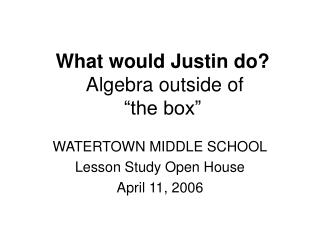DownloadDownload PresentationWhat would Justin do? Algebra outside of “the box”

# What would Justin do? Algebra outside of “the box”

Download Presentation## What would Justin do? Algebra outside of “the box”

- - - - - - - - - - - - - - - - - - - - - - - - - - - E N D - - - - - - - - - - - - - - - - - - - - - - - - - - -
##### Presentation Transcript

1. What would Justin do? Algebra outside of“the box” WATERTOWN MIDDLE SCHOOL Lesson Study Open House April 11, 2006

2. Let’s go back to Justin…

3. Calculating surface area (of a cube)

4. Justin’s way (cube)

5. Calculating surface area of a rectangular prism • S = 2lw + 2lh + 2wh

6. Justin’s way: • S = 2lw + 2lh + 2wh • S = l(2w + 2h) + 2wh

7. How did you get your answer?

8. Try to find two other correct algebraic expressions for the number of exposed faces in a prism formed by N cubes - by thinking about the problem differently.(i.e.: what would Justin do?)

9. How might you get…? • 6N –2(N –2) – 2

10. How might you get…? • 6N –2(N –2) – 2 • N+N+N+N+2

11. How might you get…? • 6N –2(N –2) – 2 • N+N+N+N+2 • 5N –2(N –2)

12. What happens if, instead of a prism that is one cube wide, we create prisms that are two cubes or three cubes wide?

13. :

14. :

15. How can you describe the exposed faces of a prism in terms of the width (W) AND the length (N)?

16. How can you describe the exposed faces of a prism in terms of the width (W) AND the length (N)? • 2WN + 2W + 2N

17. How can you describe the exposed faces of a prism in terms of the width (W) AND the length (N)? • F(W,N) = 2WN + 2W + 2N (A function of two variables?!)

18. Determine the number of exposed faces of a prism that is N cubes long, W cubes wide, and H cubes high.